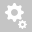##Recently viewed ticketsQuestion:

I would like to verify that the correct load factors are being applied in the load combinations.  For example, in the frequent load combination I would like to apply 0,75*MV (axle) and 0,4*MV (UDL).  In the quasi-permanent load combinations for Finland, I need to apply 0,4*MV (UDL) only.  How can I verify this in my combinations/ design/ results?

Hello ,

For a given MVL Case the psi factors are either applied for both the Tandem System and the UDL, or for neither. This is controlled from the MVL Case definition:In order to apply different factors for different combinations you will need to define different load cases. In the example you have given, you will need to define two different vehicles, one where the psi factors are 0.75 and 0.4, and one where they are 0 and 0.4. Then use each vehicle in a separate load case where the psi factors are included.

You can see the factors applied to the loads using the Results >> Moving Load Tracer, where you can choose to display the loads with the psi factors or without. Note that in both cases the analysis results consider the factors if specified in the MVL Case definition, it is only the display of the load values on screen that reflects or not the psi factor:Kind regards,

Creation date: 10/29/2018 5:47 PM (MIDAS_UK_IM)      Updated: 10/30/2018 3:19 AM (MIDAS_UK_IM)